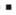Chapter 11.4, Problem 46E### Algebra and Trigonometry (MindTap ...

4th Edition
James Stewart + 2 others
ISBN: 9781305071742

#### Solutions

Chapter
Section### Algebra and Trigonometry (MindTap ...

4th Edition
James Stewart + 2 others
ISBN: 9781305071742
Textbook Problem

# 4 1 - 5 6Cramer’s Rule Use Cramer’s Rule to solve the system. { 10 x − 17 y = 21 20 x − 31 y = 39

To determine

To solve:

The system of equation {10x17y=2120x31y=39 using Cramer’s rule.

Explanation

Approach:

The Cramer’s rule for the system in two variables is given below,

The linear system of equation is given below.

{ax+by=rcx+dy=s

The linear system has the solution

x=|rbsd||abcd| and y=|arcs||abcd|

Provided that |abcd|0.

Calculation:

Consider the system of equations {10x17y=2120x31y=39.

Compare the system of equation with the standard form.

The values of a, b, c, d, r, and s are 10, 17, 20, 31, 21, and 39 respectively.

Substitute the values 10 for a, 17 for b, 20 for c, 31 for d, 21 for r, and 39 for s in the formula mentioned above of x

### Still sussing out bartleby?

Check out a sample textbook solution.

See a sample solution

#### The Solution to Your Study Problems

Bartleby provides explanations to thousands of textbook problems written by our experts, many with advanced degrees!

Get Started